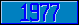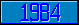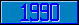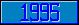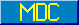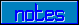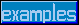## \$%PRODUCE^STRING

### M[UMPS] by Example

Approved for inclusion in a future M[UMPS] language standard as part of the library for String Handling.

This function returns a translated version of the value of its parameter.

This function could be used to reduce multiple separators to single ones:

Kill SPEC
; Two blanks will be reduced to a single one
Set SPEC(1,1)=" ",SPEC(1,2)=" "
; translate all relevant separators into blanks
Set X=\$TRanslate(X,"!?,.;:()"," ")
; and reduce multiple blanks to singletons
Set X=\$%PRODUCE^STRING(X,.SPEC)

A soundex algorithm for English names:

 1 Remove non-alpha and convert lower case to upper case 2 Examine first letters PH --> F CE --> S KN --> N WR --> R C --> K 3 Ignore all other vowels (A, E, I, O and U) 4 Then replace MP --> M ST --> S DG --> D TCH --> CH GHT --> HT 5 Then make the following equivalent B, F, P and V C, G, J, K, Q, S, X and Z D and T M and N 6 Finally, remove multiple consecutive occurrences of the same character

SOUNDEX(A) New B,C,UP,LOW,I,S1,S2
Set UP="ABCDEFGHIJKLMNOPQRSTUVWXYZ"
Set LOW="abcdefghijklmnopqrstuvwxyz"
Set A=\$TRanslate(A,LOW_"-., ()'",UP)
For I=1:1:5 Set C(I,1)=\$Piece("PH C CE KN WR"," ",I)
For I=1:1:5 Set C(I,2)=\$Piece("F. K S. N. R."," ",I)
Set I=\$%REPLACE^STRING(\$Extract(A,1,2),.C)
Set \$Extract(A,1,2)=I
Set A=\$TRanslate(A,"AEIOUY.")
For I=1:1:5 Do
. Set C(I,1)=\$Piece("MP ST DG TCH GHT"," ",I)
For I=1:1:5 Do
. Set C(I,2)=\$Piece("M. S. D. CH. HT." ",I)
Set A=\$%REPLACE^STRING(A,.C)
Set S1="BFPVCGJKQSXZDTMN."
Set S2="BBBBCCCCCCCCDDMM"
Set A=\$TRanslate(A,S1,S2)
For I=1:1:26 Do
. Set C(I,1)=\$Char(64+I,64+I)
. Set C(I,2)=\$Char(64+I)
Set A=\$%PRODUCE^STRING(A,.C)
Quit A

The MDC has approved code to approximate the return value of this function. Implementors are encouraged to provide more accurate and efficient code).